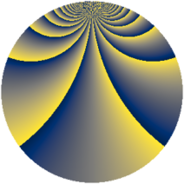# Properties

 Label 1805.2.vLevel $1805$ Weight $2$ Character orbit 1805.v Rep. character $\chi_{1805}(39,\cdot)$ Character field $\Q(\zeta_{38})$ Dimension $3384$ Sturm bound $380$

# Related objects

## Defining parameters

 Level: $$N$$ $$=$$ $$1805 = 5 \cdot 19^{2}$$ Weight: $$k$$ $$=$$ $$2$$ Character orbit: $$[\chi]$$ $$=$$ 1805.v (of order $$38$$ and degree $$18$$) Character conductor: $$\operatorname{cond}(\chi)$$ $$=$$ $$1805$$ Character field: $$\Q(\zeta_{38})$$ Sturm bound: $$380$$

## Dimensions

The following table gives the dimensions of various subspaces of $$M_{2}(1805, [\chi])$$.

Total New Old
Modular forms 3456 3456 0
Cusp forms 3384 3384 0
Eisenstein series 72 72 0

## Trace form

 $$3384 q + 148 q^{4} - 17 q^{5} - 38 q^{6} + 150 q^{9} + O(q^{10})$$ $$3384 q + 148 q^{4} - 17 q^{5} - 38 q^{6} + 150 q^{9} + 30 q^{10} - 30 q^{11} + 12 q^{14} - 29 q^{15} - 212 q^{16} - 42 q^{19} - 23 q^{20} - 58 q^{21} - 22 q^{24} - 17 q^{25} - 58 q^{26} - 90 q^{29} + 71 q^{30} - 30 q^{31} - 54 q^{34} - 21 q^{35} - 200 q^{36} - 198 q^{39} - 15 q^{40} - 30 q^{41} - 10 q^{44} - 88 q^{45} - 80 q^{46} + 122 q^{49} - 99 q^{50} - 118 q^{51} - 84 q^{54} + 15 q^{55} - 128 q^{56} - 18 q^{59} - 39 q^{60} - 26 q^{61} + 144 q^{64} - 83 q^{65} + 2 q^{66} - 252 q^{69} + 117 q^{70} - 98 q^{71} + 132 q^{74} + 243 q^{75} + 542 q^{76} - 54 q^{79} + 191 q^{80} - 274 q^{81} + 52 q^{84} - 53 q^{85} - 360 q^{86} + 96 q^{89} + 21 q^{90} - 44 q^{91} + 154 q^{94} - 58 q^{95} - 416 q^{96} - 76 q^{99} + O(q^{100})$$

## Decomposition of $$S_{2}^{\mathrm{new}}(1805, [\chi])$$ into newform subspaces

The newforms in this space have not yet been added to the LMFDB.